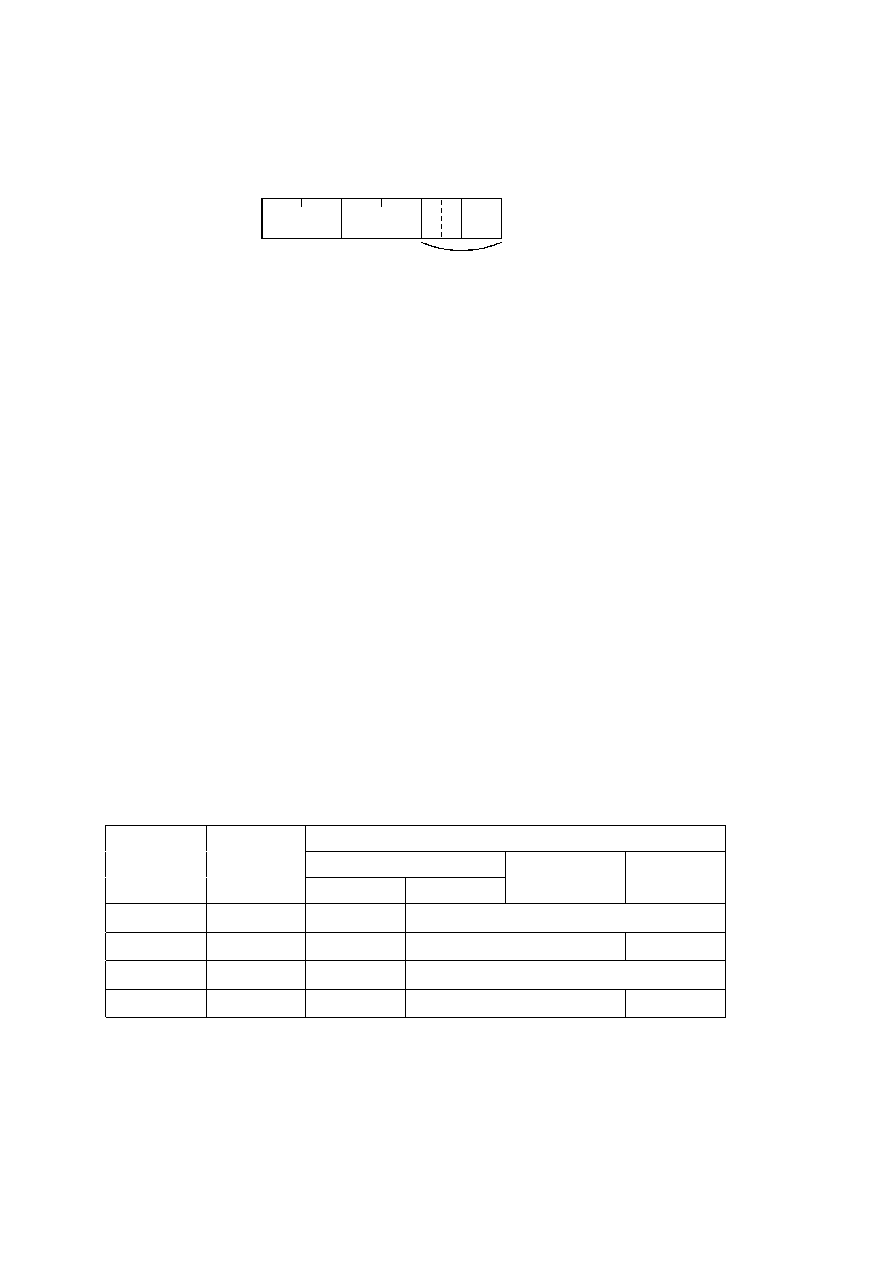ISO/IEC 10918-1 : 1993(E)
B.2.4.3
Arithmetic conditioning table-specification syntax
Figure B.8 specifies the marker segment which defines one or more arithmetic coding conditioning table specifications.
These replace the default arithmetic coding conditioning tables established by the SOI marker for arithmetic coding
processes. (See F.1.4.4.1.4 and F.1.4.4.2.1.)
Tc
DAC
La
Tb
Cs
TISO0900-93/d026
Define arithmetic conditioning segment
Multiple (t = 1, ..., n)
Figure B.8 ­ Arithmetic conditioning table-specification syntax
Figure B.8 [D26], = 3 cm = 117.%
The marker and parameters shown in Figure B.8 are defined below. The size and allowed values of each parameter are
given in Table B.6.
DAC:
Define arithmetic coding conditioning marker ­ Marks the beginning of the definition of arithmetic
coding conditioning parameters.
La:
Arithmetic coding conditioning definition length
­
Specifies the length of all arithmetic coding
conditioning parameters shown in Figure B.8 (see B.1.1.4).
Tc:
Table class ­ 0 = DC table or lossless table, 1 = AC table.
Tb:
Arithmetic coding conditioning table destination identifier ­ Specifies one of four possible destinations at
the decoder into which the arithmetic coding conditioning table shall be installed.
Cs:
Conditioning table value ­ Value in either the AC or the DC (and lossless) conditioning table. A single
value of Cs shall follow each value of Tb. For AC conditioning tables Tc shall be one and Cs shall contain a
value of Kx in the range 1
Kx
63. For DC (and lossless) conditioning tables Tc shall be zero and Cs shall
contain two 4-bit parameters, U and L. U and L shall be in the range 0
L
U
15 and the value of Cs shall be
L
+
16
×
U.
The value n in Table B.6 is the number of arithmetic coding conditioning tables specified in the DAC marker segment.
The parameters L and U are the lower and upper conditioning bounds used in the arithmetic coding procedures defined
for DC coefficient coding and lossless coding. The separate value range 1-63 listed for DCT coding is the Kx conditioning
used in AC coefficient coding.
Table B.6 ­ Arithmetic coding conditioning table-specification parameter sizes and values
Values
Parameter
Size (bits)
Sequential DCT
Progressive DCT
Lossless
Baseline
Extended
La
16
Undefined
2
+
2
×
n
Tc
1
4
Undefined
0, 1
0
-255
Tb
1
4
Undefined
0-3
Cs
1
8
Undefined
0-255 (Tc = 0), 1-63 (Tc = 1)
0-255
42
CCITT Rec. T.81 (1992 E)